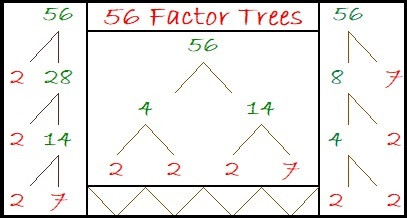# 909 and Level 4

909 is a palindrome in base 10 because 9(100) + 0(10) + 9(1) = 909.

It is also palindrome 757 in BASE 11 because 7(121) + 5(11) + 7(1) = 909.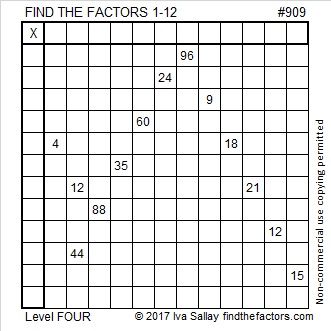Print the puzzles or type the solution on this excel file: 12 factors 905-913

Here’s a little more about the number 909:

30² + 3² = 909

909 is the hypotenuse of Pythagorean triple 180-891-909, which is 9 times (20-99-101) and can be calculated from 2(30)(3), 30² – 3², 30² + 3²

As you know, 909 is made with 9’s and 0’s. OEIS.org informs us that 909 times 2, 3, 4, 5, 6, 7, 8, or 9 do NOT contain even one 9 or 0. That’s a little spooky, but see for yourself: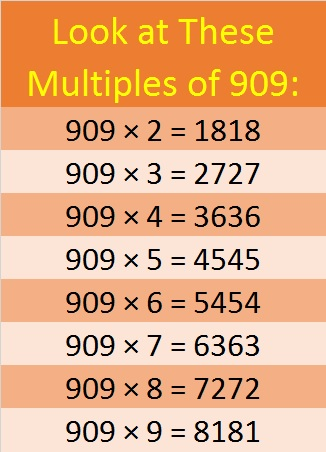• 909 is a composite number.
• Prime factorization: 909 = 3 × 3 × 101, which can be written 909 = 3² × 101
• The exponents in the prime factorization are 2 and 1. Adding one to each and multiplying we get (2 + 1)(1 + 1) = 3 × 2  = 6. Therefore 909 has exactly 6 factors.
• Factors of 909: 1, 3, 9, 101, 303, 909
• Factor pairs: 909 = 1 × 909, 3 × 303, or 9 × 101
• Taking the factor pair with the largest square number factor, we get √909 = (√9)(√101) = 3√101 ≈ 30.14962686# 668 and Level 3

668 is the sum of consecutive prime numbers 331 and 337.

68 is divisible by 4 so 668 and every other number ending in 68 is divisible by 4.

80 + 81 + 82 + 83 + 84 + 85 + 86 + 87 = 668Print the puzzles or type the solution on this excel file: 12 Factors 2015-11-02

—————————————————————————————————

• The first ten multiples of 668 are 668, 1336, 2004, 2672, 3340, 4008, 4676, 5344, 6012, and 6680.
• 668 is a composite number.
• Prime factorization: 668 = 2 x 2 x 167, which can be written 668 = (2^2) x 167
• The exponents in the prime factorization are 2 and 1. Adding one to each and multiplying we get (2 + 1)(1 + 1) = 3 x 2  = 6. Therefore 668 has exactly 6 factors.
• Factors of 668: 1, 2, 4, 167, 334, 668
• Factor pairs: 668 = 1 x 668, 2 x 334, or 4 x 167
• Taking the factor pair with the largest square number factor, we get √668 = (√4)(√167) = 2√167 ≈ 25.845696.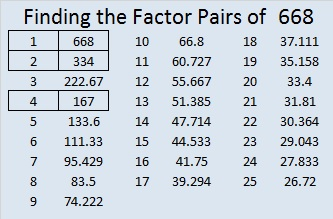—————————————————————————————————

A Logical Approach to solve a FIND THE FACTORS puzzle: Find the column or row with two clues and find their common factor. (None of the factors are greater than 12.)  Write the corresponding factors in the factor column (1st column) and factor row (top row).  Because this is a level three puzzle, you have now written a factor at the top of the factor column. Continue to work from the top of the factor column to the bottom, finding factors and filling in the factor column and the factor row one cell at a time as you go.# 163 and Level 3

• 163 is a prime number.
• Prime factorization: 163 is prime.
• The exponent of prime number 163 is 1. Adding 1 to that exponent we get (1 + 1) = 2. Therefore 163 has exactly 2 factors.
• Factors of 163: 1, 163
• Factor pairs: 163 = 1 x 163
• 163 has no square factors that allow its square root to be simplified. √163 ≈ 12.7671How do we know that 163 is a prime number? If 163 were not a prime number, then it would be divisible by at least one prime number less than or equal to √163 ≈ 12.8. Since 163 cannot be divided evenly by 2, 3, 5, 7, or 11, we know that 163 is a prime number.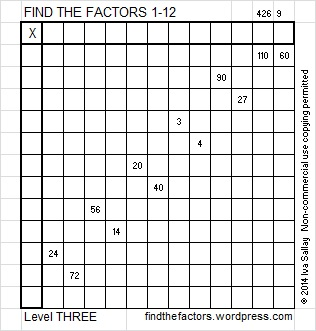Excel file of puzzles and previous week’s factor solutions: 12 Factors 2014-06-30

A Logical Approach to FIND THE FACTORS: Find the column or row with two clues and find their common factor. Write the corresponding factors in the factor column (1st column) and factor row (top row).  Because this is a level three puzzle, you have now written a factor at the top of the factor column. Continue to work from the top of the factor column to the bottom, finding factors and filling in the factor column and the factor row one cell at a time as you go.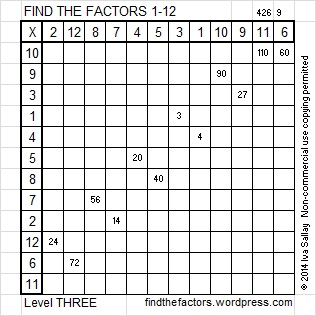# 75 and Level 6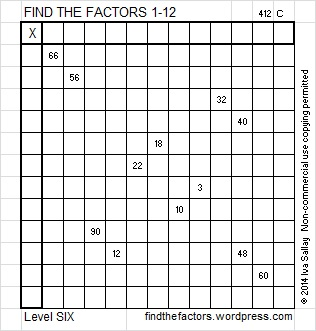This week’s puzzles for you to solve

75 is a composite number. 75 = 1 x 75, 3 x 25, or 5 x 15. Factors of 75: 1, 3, 5, 15, 25, 75. Prime factorization: 75 = 3 x 5 x 5, which can also be written 75 = 3 x 5².75 is never a clue in the FIND THE FACTORS puzzles.

75 is in this wonderful pattern. Can you tell what the pattern is?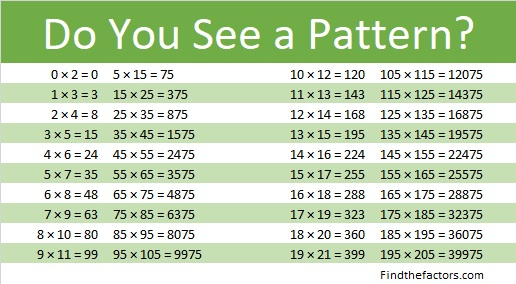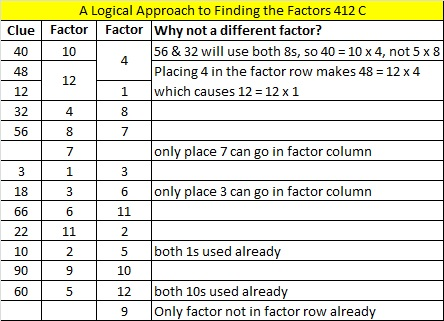# 74 and Level 5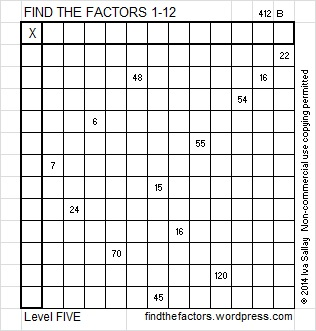This week’s puzzles for you to solve

74 is a composite number. 74 = 1 x 74 or 2 x 37. Factors of 74: 1, 2, 37, 74. Prime factorization: 74 = 2 x 37.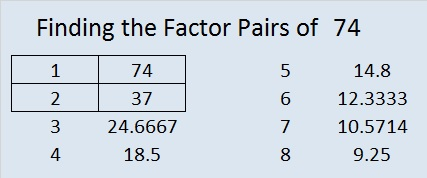74 is never a clue in the FIND THE FACTORS puzzles.# 60 and Pay No Attention to That Man Behind the Curtain

### Today’s Puzzle:

When solving a level 6 puzzle, trying to place the numbers from 1 to 10 in the top row as well as the first column can be frustrating. Every single clue appears to have more than one possible answer. How can someone guess right with every single number placement? Is seeing the answer the only way to solve the puzzle?

Here is this week’s level 6 puzzle. Usually, to solve a puzzle, you start with a row or column with 2 clues.  There are 6 rows or columns with more than one clue, and every one of them is no help!You can also find this and a few other puzzles in an excel file here. If you have a spreadsheet program on your computer, you can access it. If you enable editing in excel, you can type your answers directly onto the puzzle, and you can also easily print the puzzles.

### A Peek at the Possible Factors for Each of Last Week’s Clues:

“Pay no attention to the that man behind the curtain!” is a famous line from The Wizard of Oz.  Everyone in Oz thought the Wizard was powerful enough to solve any problem. When Dorthy and her friends looked behind the curtain they discovered that he was really an ordinary man who was able to find an advantage in what other people didn’t know. It turns out that other people actually had the abilities they thought only the Wizard had. Today we will take a peek behind the curtain of a level 6 puzzle and discover that it really isn’t as intimidating as we might imagine and that you have the ability to solve such a puzzle, too. This is what last week’s level 6 puzzle looks like from behind the curtain: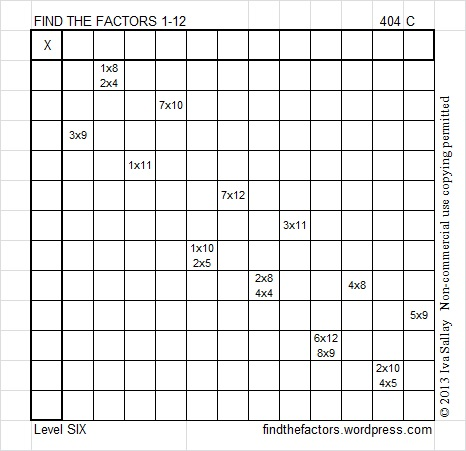Many people try to start with the row that contains the clue 4 x 8 = 32. (When I solve this puzzle, that row is actually the one I FINISH with!) Instead, look at the row with no clues. If you study all the clues in the puzzle, you will notice that 6 is listed as a factor only once. If there were a clue in the row without any clues, that clue would be a multiple of 6. That hint will keep you going for several clues. It also eliminates 8 as a possible factor of 72 and ensures that 1 x 8, not 2 x 4, must be used for the clue 8. This means that 2 x 5, not 1 x 10, must be used for the clue 10 because both ones will have been used. That means that 2 x 10, not 4 x 5, must be used for the clue 20 because the number 10 has to be used as a factor twice. Here is the completed puzzle:### Factors of 60:

60 is a composite number. 60 = 1 x 60, 2 x 30, 3 x 20, 4 x 15, 5 x 12, or 6 x 10. Factors of 60: 1, 2, 3, 4, 5, 6, 10, 12, 15, 20, 30, 60. Prime factorization: 60 = 2 x 2 x 3 x 5, which can also be written 60 = 2² x 3 x 5.Sometimes 60 is a clue in the FIND THE FACTORS puzzles. Even though it has many other factors, we only use 6 x 10 for the FIND THE FACTORS 1-10 puzzles and 6 x 10 or 5 x 12 for the FIND THE FACTORS 1-12 puzzles.

### Sum-Difference Puzzle:

60 has six factor pairs. One of those factor pairs adds up to 17, and another one subtracts to 17. If you can identify those factor pairs, then you can solve this puzzle:# 59 and Valentine Gif

• 59 is a prime number.
• Prime factorization: 59 is prime.
• The exponent of prime number 59 is 1. Adding 1 to that exponent we get (1 + 1) = 2. Therefore 59 has exactly 2 factors.
• Factors of 59: 1, 59
• Factor pairs: 59 = 1 x 59
• 59 has no square factors that allow its square root to be simplified. √59 ≈ 7.6811How do we know that 59 is a prime number? If 59 were not a prime number, then it would be divisible by at least one prime number less than or equal to √59 ≈ 7.7. Since 59 cannot be divided evenly by 2, 3, 5, or 7, we know that 59 is a prime number.

59 is never a clue in the FIND THE FACTORS puzzles.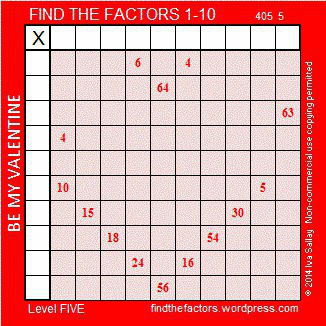Valentine’s Day is almost here. Here is a Valentine you can share with your friends who enjoy solving puzzles.

To solve the puzzle, the numbers 1 through 10 need to be written on the top row and also on the first column so that those numbers and the clues inside the puzzle make a multiplication table. Since it’s a level 5 puzzle, it may be a little challenging.

Your friend will not have to wait a whole week for the solution because there is a gif that will solve it quickly and logically. The gif could be the valentine, too.

This week’s puzzles are available in an excel file here. If you have a spreadsheet program on your computer, you can access it. If you enable editing in excel, you can type your answers directly onto the puzzle, and you can also easily print the puzzles.

Here are the factors to last week’s puzzle.<a data-pin-do=”embedPin” href=”http://www.pinterest.com/pin/506092076847813688/”></a&gt;
<!– Please call pinit.js only once per page –>
//assets.pinterest.com/js/pinit.js

# 58 and OXOXOXOXOXOXOX

58 is a composite number. 58 = 1 x 58 or 2 x 29. Factors of 58: 1, 2, 29, 58. Prime Factorization: 58 = 2 x 29.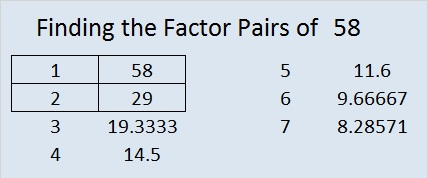58 is never a clue in the FIND THE FACTORS puzzles.Hugs and Kisses, OXOXOXOXOXOXOX, these were some of my stepmother’s favorite things. This week in anticipation of Valentine’s Day next week, I’ve included both an X puzzle and this O puzzle. Valentine’s Day was also my dad’s and my stepmother’s wedding anniversary, and she always made sure it was a special day for everyone who lived in our house. I’m sure that you also have many happy Valentine’s Day memories.

To solve this O puzzle, consider the clues given in the puzzle. Then write the numbers 1-12 in the top row and again in the first column so that the puzzle becomes a multiplication table.

This week’s puzzles are available in an excel file here. If you have a spreadsheet program on your computer, you can access it. If you enable editing in excel, you can type your answers directly onto the puzzle, and you can also easily print the puzzles.

Here are the factors for last week’s level 4 Puzzle:Here is one way those factors could be found:# 57 and Cupid’s arrow is on its way!

57 is a composite number. 57 = 1 x 57 or 3 x 19. Factors of 57: 1, 3, 19, 57. Prime factorization: 57 = 3 x 19.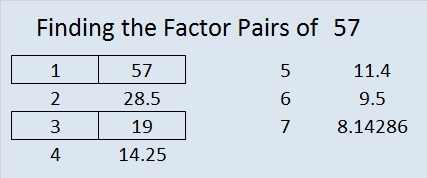57 is never a clue in the FIND THE FACTORS puzzles.

How do you feel about factoring?

If you will let Cupid hit you with this arrow, you might just fall in love with factors.

Factoring is very important in many levels of mathematics, so enjoying it can be a very good thing.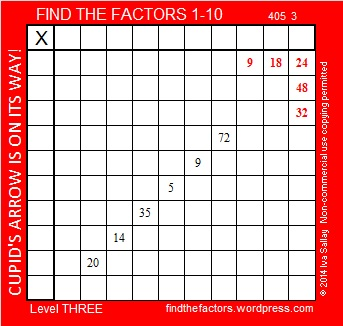I love factoring. I hope you will let Cupid’s arrow hit you so you will love factoring, too.

This week’s puzzles are available in an excel file here. If you have a spreadsheet program on your computer, you can access it. If you enable editing in excel, you can type your answers directly onto the puzzle, and you can also easily print the puzzles.

The factors for last week’s level 3 Puzzle:Here’s how last week’s puzzle was solved:# 56 and Level 2

56 is a composite number. 56 = 1 x 56, 2 x 28, 4 x 14, or 7 x 8. Factors of 56: 1, 2, 4, 7, 8, 14, 28, 56. Prime factorization: 56 = 2 x 2 x 2 x 7, which can also be written 56 = 2³ x 7.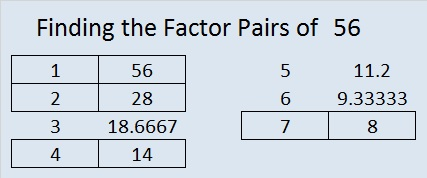Sometimes 56 is a clue in the FIND THE FACTORS puzzles. Even though it has other factors, the only multiplication fact we use is 7 x 8 = 56.This week’s puzzles are available in an excel file here. If you have a spreadsheet program on your computer, you can access it. If you enable editing in excel, you can type your answers directly onto the puzzle, and you can also easily print the puzzles.

The factors for last week’s level 2 Southeast Arrow Puzzle:Here are three different factor trees for the number 56: